## Main

The Berry curvature is a geometrical property of an energy band, which plays a key role in many physical phenomena as it encodes how eigenstates evolve as a local function of parameters1. In a two-dimensional (2D) quantum Hall system, for example, the integral of the Berry curvature over the 2D Brillouin zone determines the Chern number: a global topological invariant that underlies the quantization of Hall transport for a 2D filled energy band2. As first explained by Thouless3, there can be an analogous topological quantization of particle transport in a 1D band insulator, when the lattice potential is ‘pumped’, that is, is slowly and periodically modulated in time. Also in this case, the geometrical and topological properties are defined for an effective 2D parameter space, but now one spanned by the 1D Bloch momentum and the external periodic pumping parameter.

A local non-zero Berry curvature can have striking physical effects in both 2D systems and 1D pumps, regardless of whether the global topological Chern number is non-trivial. In the simplest case, a semi-classical wavepacket moves as a coherent object governed by classical equations of motion with an additional ‘anomalous’ Hall velocity due to the geometrical Berry curvature at its centre-of-mass, as an external force is applied or as the control parameter is pumped1,4. As highlighted further below, this anomalous transport can be understood physically as the Berry curvature acting like a magnetic field in parameter space5,6,7.

In recent years, there have been many landmark experiments to engineer and study geometrical and topological energy bands in ultracold gases and photonics8,9,10,11,12,13,14,15,16,17,18,19,20,21,22,23,24,25,26,27,28. In photonics, for example, topological edge states have been studied in a wide variety of (effectively) 1D set-ups, such as quantum walks14 and pumping in optical quasicrystals13,29,30, as well as in 2D quantum Hall-like systems of photonic crystals9,10,11, propagating waveguides12 and silicon ring resonators15,16. However, while the existence of such edge states is linked to the non-trivial global topological properties of bulk bands, a direct measurement of the Berry curvature itself has so far not been realized. In ultracold atoms, instead, there has been much progress in this direction, for example, with experiments exploiting the effects of Berry curvature on particle transport to extract, in 2D systems, the Chern number19 and topological phase diagrams20, and, in 1D systems, both the quantized ‘Thouless pumping’ of atoms in a filled band23,24 and the un-quantized ‘geometrical pumping’ of a Bose–Einstein condensate25.

In this Letter, we go beyond previous experiments to provide the first direct measurement of the Berry curvature in an optical system. We also demonstrate the first full tomography of the Berry curvature of a geometrical energy band from measurements of the anomalous displacement of a coherent classical wavepacket. This approach complements existing methods in ultracold gases to measure the Berry curvature via band projection26 or using Wilson lines27, with the significant advantage that it does not require a full reconstruction of the band eigenstates as an intermediate step. Consequently, our method can be easily applied to complex systems with many bands and degrees of freedom, where a full determination of the eigenstates would be experimentally challenging.

In our ‘photonic mesh lattice’ set-up31,32, pulses are injected into two coupled optical fibre loops, so that the temporal evolution of the outgoing pulse-train envelope simulates a wavepacket in a 1D lattice. More specifically, the position of the particle is encoded in the time at which a light pulse completes a round trip through one fibre loop. Tunnelling between time slots is achieved as the two coupled loops have slightly different lengths, allowing the pulse to ‘hop’ from one time slot to another. We then apply a periodic phase modulation in one loop to engineer Floquet quasi-energy bands, in which the eigenstates depend on both the 1D ‘Bloch momentum’, Q, and on a control parameter, φ, given by the amplitude of the phase modulation. With respect to these Floquet bands, the Berry curvature takes the form1where |ψj〉 are the eigenstates making up the jth band in the effective 2D parameter space. By preparing a wavepacket with a narrow spectral width and a well-defined centre-of-mass momentum Q, we realize a geometrical charge pump by slowly ramping the phase amplitude, φ, in time. Due to this pumping, the wavepacket moves along the 1D ‘photonic mesh lattice’, with an anomalous velocity contribution1,23,24,25 due to the Berry curvature Ωjφ(t), Q. Our high-resolution observation of the wavepacket dynamics then allows us to map out the Berry curvature for an optical energy band for the first time. By bringing geometrical energy bands into the new setting of photonic mesh lattices, our work opens up new opportunities for engineering novel systems, combining topology, nonlinearity, gain or loss, which may then be studied via wavepacket dynamics.

In our experiment, we employ a time-multiplexing, linear optical scheme based on two coupled fibre loops of different lengths31,32 as shown in Fig. 1a. At the beginning of each measurement, an optical pulse with a wavelength λ = 1,555 nm is injected into the longer loop before being split by a 50/50 coupler into one pulse in each loop. After every round trip, each pulse is again split into two, although due to the different loop lengths, the short-loop pulse completes the round trip in a time, T2, which is shorter than that taken in the long loop, T1. Crucially, the average loop-length is much larger than the difference in loop lengths; in particular, the former is about 1 km, corresponding to a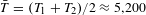ns average round trip time, while the latter is only about 7 m, corresponding to a time difference of ΔT = T1T2 ≈ 35.4 ns between round trips in the long and short loops. Furthermore, to avoid any overlap between distinct pulses, the pulse width Tpulse is set to 25 ns, such that it is shorter than the minimum pulse separation ΔT. Thanks to the separation of all of these timescales, we observe clearly separated sequences of pulses over time (see Fig. 1), so that each pulse can be straightforwardly labelled by two integers (m, n), with m being the total number of round trips and n counting how many more round trips were made in the long compared with the short loop. Of course, this picture is restricted to sequences shorter than the round trip time in order for the assignment of integers to be unambiguous; this limits the maximum number of pulses in a given sequence to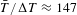.

To detect the average intensities of pulses in both loops, we use a combination of a photodiode and a digital sampling oscilloscope with a bandwidth of 200 MHz and a temporal distance of the samples of 0.4 ns. Thanks to the long pulse-width, Tpulse, dispersive effects in the optical fibres are typically unimportant, even over 400 round trips, corresponding to 400 km of propagation. However, for removing noise and for modulating the amplitude and phase of the pulses, band-pass filters and modulators are needed, as discussed in detail in the Supplementary Information. These additional components introduce losses on the order of approximately 8 dB per round trip, which are compensated by erbium-doped fibre amplifiers. In general, the use of these amplifiers destroys quantum correlations; however, all experiments here are performed with a classical, coherent bright light pulse and do not rely on quantum effects. Besides compensating any losses, the erbium-doped fibre amplifiers also allow for the use of pulses with a peak power of up to 200 mW. In the future, when combined with dispersion-compensating fibres, this system can be used, therefore, for the measurement of nonlinear effects, for example, the formation of solitons based on nonlinear self-phase modulation, which will be of great interest to study in the context of geometrical or topological energy bands. Additionally, this experimental platform also allows for the investigation of the topology of non-Hermitian systems, by including gain and loss in a controlled way. This can be achieved, by using fibre-coupled acousto-optical modulators, which are set to a transmission of 50%. Depending on an electrical radiofrequency signal, this value can be dynamically increased or decreased resulting in an effective gain or loss 32.

Based on the labelling of pulses according to (m, n), the evolution of light can be mapped to a 1 + 1-dimensional lattice as depicted in Fig. 1b, c, where m is the number of discrete time steps on the vertical axis, and n is the position along the horizontal axis, which can either increase or decrease by one at each time step. In our set-up, the evolution between each time step is: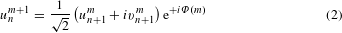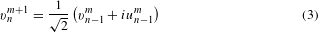where unm and vnm denote the pulse amplitudes incident on the beamsplitter from the short and long loops. As can be seen in these equations, pulse amplitudes at the m + 1 time step are an equal superposition of pulses at the previous time step m, with a relative π/2 phase shift for light that couples from one loop to the other, due to the 50/50 beamsplitter coupling the two loops. As mentioned above, we have neglected here dispersion effects and assumed that the pulse shape does not depend on the specific route taken. In the short loop, we have inserted an additional phase modulator that provides a time-dependent phase shift Φ(m). A suitable choice of this phase shift will generate the non-trivial geometrical Berry curvature as discussed in the following and in the Supplementary Information.

Without the phase shift Φ(m), the lattice depicted in Fig. 1b and described by the above equations (2) and (3) is periodic under a double step in the time step mm + 2 and the position nn + 2. Guided by the tight-binding analogy above, we apply the so-called Floquet–Bloch ansatz for the eigenstates32,33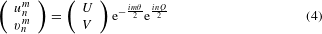where the parameter Q is the ‘Bloch momentum’ along the 1D lattice, introduced above, and θ is the propagation constant or ‘quasi-energy’. Here, the periodic part of the eigenstates in the jth band is denoted by ψj = (U, V)T and the quasi-energy band structure satisfies33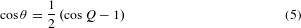leading to two bands that are periodic in both Q and θ as expected in a Floquet system, with a band-touching at the edge of the 1D Brillouin zone where Q = ±π (see Supplementary Note 2). In general, the number of bands can be increased by using a ‘spatially’-structured phase modulation, that is, a modulation dependent on position n, which increases the size of the unit cell32, or by adding additional fibre loops of different length, which can also increase the effective ‘spatial’ dimensionality.

We can engineer the time-dependent phase shift Φ(m) to realize energy bands with non-trivial geometrical properties, by, for example, cyclically applying a positive phase +φ for even time steps and −φ for odd (see Fig. 2a), and hence breaking a symmetry of the 1D lattice (see Supplementary Methods for further discussion). Experimentally, this means switching the sign of the phase modulation every time that all pulses complete another round trip. Therefore, the necessary modulation frequency of the phase modulator is on the order of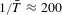kHz. As the double-step periodicity is maintained, we again use the above ansatz equation (4) and find two bands satisfying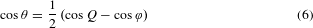as plotted in Fig. 2b, c (see also Supplementary Information). The manifold of eigenstates is now defined over the Bloch momentum Q and the periodic phase amplitude φ, allowing us to define the Berry curvature in equation (1). As shown in Fig. 2d, this Berry curvature is singular at points where the two bands touch but otherwise can take large non-zero positive and negative values across the effective 2D parameter space.

To probe the Berry curvature, we prepare an optical wavepacket by exciting a superposition of states in the jth band with a well-defined mean Bloch momentum Q and with a narrow momentum spread ΔQ ≈ 0.05π. This can be achieved in good approximation by initially generating a chain of pulses with a Gaussian envelope in the short and long loop, and by setting the correct phase modulation between the envelopes in both loops34. Then, by slowly increasing the magnitude of the phase modulation after every second time step, we ‘pump’ the wavepacket along the band in the effective 2D parameter space. We distinguish therefore between two timescales; firstly, there is the fast timescale m, which counts the round trips and over which the sign of the phase flips as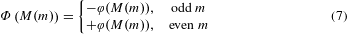while the phase magnitude φ, that is, the pumping parameter, is determined by the slower timescale M(m). Here, we let the phase magnitude increase linearly with M as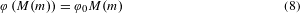and set M(m) = m/2. For simplicity, hereafter in our notation we drop the explicit dependence on m. Note that the fast phase variation in equation (7) is directly included in the bands thanks to the ansatz in equation (4), while the slow variation in equation (8) adiabatically ‘pumps’ the system, provided that the rate φ0 is sufficiently small compared with the bandgap.

The centre-of-mass of the Gaussian envelope then evolves along the 1D lattice with a total velocity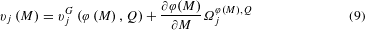where the first term is the usual group velocity from the band dispersion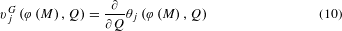and the second is the anomalous velocity due to the Berry curvature. This is easiest to understand for a wavepacket in a 2D lattice, in which case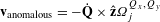, with the Berry curvature,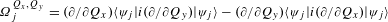playing the role of a magnetic field in the 2D Brillouin zone, which is defined over the Bloch momenta Qx and Qy (refs 5,6,7). This anomalous velocity is then the momentum-space analogue of the Lorentz force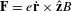, acting on a charged electron in a real-space magnetic field,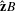, perpendicular to the 2D lattice. In a 1D pump, Qy is replaced by the periodic pump parameter, in a procedure often known as dimensional reduction35. As the pump parameter is externally controlled, the dynamics along one direction are frozen out, leaving only the anomalous term in equation (9).

Experimentally, what we measure is the shift in the centre-of-mass of the Gaussian envelope along the ‘position’ n as a function of the number of modulation periods M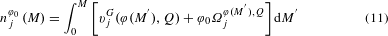We note that the limit of topological Thouless pumping can be recovered from this expression, if we consider the motion of a filled and gapped energy band after a complete adiabatic pump cycle. Then, the shift is quantized as an integer3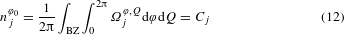where BZ stands for Brillouin zone, Cj is the topological Chern number, and where we have used that the group velocity integrates to zero over the full 2D parameter space. In our experiment, however, we consider a system in which the energy bands are not gapped and so the assumption of adiabatic evolution across the entire 2D parameter space breaks down and equation (12) is not applicable. Rather, we focus here on the local Berry curvature, and, in particular, on ways to exploit the local sensitivity of a spectrally narrow wavepacket to experimentally extract its value.

To cleanly separate Berry curvature from group velocity effects, we apply a variant of the ‘time-reversal’ protocol36 and compare the propagation (see Fig. 3a) under a reversal of the sign of the phase magnitude (that is, +φ0 and −φ0) to find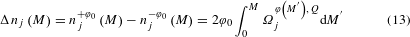where we have used that, for this modulation, vjG(φ, Q) = vjG(−φ, Q) and Ωjφ, Q = Ωjφ, Q (see Supplementary Information 4 and 5). By measuring the real-space propagation of the wavepackets for ±φ0, the dynamical evolution and hence the influence of the non-vanishing Berry curvature is directly recorded. After exciting an initial eigenstate, no further information about the eigenstates is required as we directly measure the Berry curvature from its physical consequences on wavepacket dynamics; this is in contrast to other approaches for measuring the Berry curvature26,27 that require a full reconstruction of the band eigenstates as an intermediate step. The integrated Berry curvature over a complete phase-pumping cycle is shown in Figs 2e and 3b. Note that this anomalous displacement can become very large as we approach the band-touching points where the Berry curvature diverges and adiabaticity breaks down; experimentally we measure an anomalous displacement of up to approximately 1 position over 200 time steps for a wavepacket centred around Q = 0.1π subject to a phase gradient of φ0 = π/50. The general applicability of this method is further confirmed in Supplementary Information 8, where the same experimental protocol is applied to a second example of modulation and pumping sequence.

As the most important advance of our work, the wavepacket evolution can be measured after each discrete time step, allowing for unprecedented high time resolution of the dynamics. We exploit this to map out the local Berry curvature directly from anomalous transport for the first time, by performing a discrete differentiation of the lateral shift Δnj(M) as (see Fig. 3c)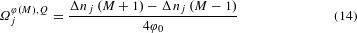The result of this procedure is shown in Fig. 3d, e; as can be seen, the main features of the Berry curvature are well captured, such as the overall symmetry and sign, the quantitative magnitude over much of the band and the predicted complementarity of the two bands1,26 (that is, Ω1φ, Q = −Ω2φ, Q). Due to the ‘on the fly’ tracking of the wavepacket, we can measure the Berry curvature even along trajectories that eventually hit band singularities. Close to the centre of the Brillouin zone (Q ≈ 0), for example, we can extract the Berry curvature until the singularity is hit at φ = π; afterwards, part of the population is transferred to the other band and the measurement is no longer meaningful. For a gapped band, this technique would straightforwardly give the topological Chern number (equation (12)) when we integrate the measured Berry curvature over the effective 2D parameter space.

In conclusion, we have demonstrated that anomalous wavepacket dynamics can provide an important experimental tool for mapping out the local geometrical properties of energy bands. This versatility of this scheme should allow it to be applied in a variety of configurations, including systems with gain and loss32 and/or non-trivial topological invariants. In the presence of nonlinearities, this technique could provide a new approach for the creation and manipulation of different wavepacket structures, such as solitons. Given its simplicity, our experimental set-up also holds the promise of transferring geometrical and topological concepts into the applied world of optoelectronics.

## Methods

A detailed description of the experimental platform is included in Supplementary Note 1.

To reduce the noise limit of the data acquisition, the measured propagation was averaged over approximately 25 realizations. For each band, we sequentially prepared wavepackets with an initial mean Bloch momentum Q, which was scanned from −π to π in steps of 0.05π. For each value of Q, we performed measurements for −φ0 and +φ0 before then setting a new value of Q.

To start with a spatially broad and thus spectrally narrow distribution at a specific Bloch momentum Q, a single seed pulse is injected into the fibre loop system. By switching off one of the two loops in a cyclic fashion after every second time step m, the system is converted into a lossy mesh lattice as discussed in ref. 37. The resulting propagation is given by a diffusion equation leading to a coherent splitting of the initial pulse into a chain of pulses with a Gaussian envelope. Due to this diffusive motion, the width of the resulting wavepacket grows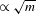. Thus, for 200 time steps a wavepacket with a width of ≈8 positions with respect to the full-width at half-maximum of the intensity is generated, which provides a sufficiently small spectral width ΔQ ≈ 0.05π to map out the local Berry curvature.

To excite a specific Bloch momentum Q, we apply a phase of φ = Q during the diffusive motion and a phase of φ = (Q ± π)/2 for the last time step before the actual propagation. The choice of the sign allows for a selection of either the upper or the lower band. As discussed in refs 34,37, in this way, the appropriate eigenstate corresponding to a Bloch momentum Q is excited. After the preparation of the Gaussian beam over the first 200 time steps, there is no further switching-off of either loop and, instead, we measure the wavepacket dynamics during the next 200 time steps, using the phase modulator to adiabatically ‘pump’ the system as discussed in the main text.

The centre-of-masses of the wavepackets were extracted by fitting Gaussian distributions to the cross-sections for each time step. A detailed explanation of this fitting procedure, together with examples, is included in Supplementary Note 6.

The error bars depicted in Fig. 2e are estimated by the spectral width ΔQ ≈ 0.05π of the initial excitation in the horizontal direction and by the uncertainty of the fit procedure for a 95% confidence level in the vertical direction.

For the full reconstruction of the Berry curvature, it is necessary to differentiate the measured integrated Berry curvature with respect to the time step m as discussed in equation (14). Directly before evaluating the derivative, a moving average filter is applied to the data to decrease the noise level. A span of 5 values was chosen for the averaging. Raw data without averaging are provided in the Supplementary Methods.

### Data availability.

The data that support the plots within this paper and other findings of this study are available from the corresponding author on request.